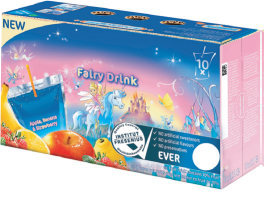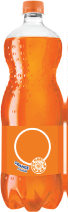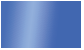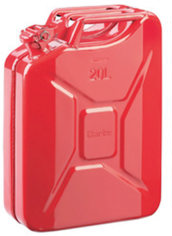# Lesson Notes By Weeks and Term - Primary 3

Capacity

Week 4

Subject: Mathematics

Class: Primary 3

Term: 3rd Term

Topic:  Capacity

The amount of liquid a container holds is called its capacity.

Liquids are things like: water, milk, kerosene, oil, petrol, juice, mineral water, etc.

The standard measure for liquids is the litre l and millilitre mlLiquids in large containers are measured in litres.

Petrol and diesel are measured in litres.

Liquids in small containers are measured in millilitres.

Syrups, lotions and perfumes are measured in millilitres.

Exercise

1. Name five liquids measured in litres.
2. Name five liquids measured in millilitres.

Activity

Check the empty containers of liquids you used in your home. Bring in one that

has 1 litre written on it. Compare with your friends’ containers. Do they all have the

same shape?

Capacity of containers

Note

10 millilitres (ml) = 1 centilitre (cl)

10 centilitres (cl) = 1 decilitre (dl)

10 decilitres (ôô) = 1 litre (l)

1000 litres = 1 kilolitre (kl)

Exercise 1

1. How many litres or millilitres can each contain? Use a litre jug to measure.

Container                 Measurement in litres

1. Bucket

2. Cooking pot

3. Plastic basin

4. Big jerry can

5. Small jerry can

6. Jug

7. Mug

8. Water dispenser jar

9. Pure water sachetExamples

This shows us how many 1/2litres there are in 1 1/2litres.

Solution

11/2litres = 1 litre + 1/2litre

1 litre = 2 one-half litres

Therefore, 11/2litres = 3 one-half litres

This shows us how many 1/4litres there are in 3 litres.

Solution

3 litres              = 1l + 1l + 1l

1 litre                = 4 one-quarter litres

Therefore, 3 litres = 3 × 4 one-quarter litres = 12 one-quarter litres

Exercise 2

1. How many 1/2 litres are there in:

a) 3 litres b) 2 1/2litres c) 4 litres d) 6 litres?

2. How many 1/4litres are there in:

a) 11/2litres b) 4 litres c) 3 litres d) 21/4litres?

Examples

1 litre + 5 litre = 6 litres

1L + 5l = 6l

20 litres – 15 litres = 5 litres

40ml + 30ml = 70 ml

150ml – 130ml = 20ml

Exercise

1. 170 ml + 250ml 2. 255ml + 175ml
2. 30 litres + 135 litres 4. 71 litres + 52 litres.

B. Subtract the following.

1. 6l – 4l 2. 13l – 5L   3. 270ml – 135 ml    4. 500ml – 125ml
2. Change to litres and millilitres.
3. 1200ml = 1 000 ml + 200mln = 1l 200ml
4. 1500ml = 3. 7500ml = 4. 4400 ml =
5. 3200ml = 6. 4750ml =
6. Change to millilitres only.
7. 1l 250ml = 1l + 250ml = 1000ml + 250ml = 1250ml
8. 8l 330ml = 3. 9l  200ml  = 4. 5l  950 ml =
9. 8l 750ml = 6. 7l  305ml =

Problems involving capacity

Exercise

Solve the problems. The first has been done for you.

1. Mr Salaam has 12 litres of fuel in his tank. He bought 25 litres. How many litresof fuel are in the tank now?

Mr Salaam bought 12 litres

+25 litres

37 litres

and he bought

Altogether, he bought